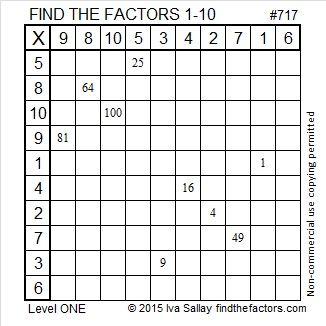# 717 and Level 1

• 717 is a composite number.
• Prime factorization: 717 = 3 x 239
• The exponents in the prime factorization are 1 and 1. Adding one to each and multiplying we get (1 + 1)(1 + 1) = 2 x 2 = 4. Therefore 717 has exactly 4 factors.
• Factors of 717: 1, 3, 239, 717
• Factor pairs: 717 = 1 x 717 or 3 x 239
• 717 has no square factors that allow its square root to be simplified. √717 ≈ 26.77685567799.If you know a few square numbers, this puzzle is pretty easy:Print the puzzles or type the solution on this excel file: 10 Factors 2015-12-21

—————————————————————————————

Here are a few more facts I’ve found about the number 717:

717 can be written as the sum of consecutive numbers 2 ways:

• 358 + 359 = 717; that’s 2 consecutive numbers.
• 238 + 239 + 240 = 717; that’s 3 consecutive numbers.

717 is a palindrome in three bases:

• 1011001101 BASE 2
• 717 BASE 10
• 393 BASE 14

——————————————————————————————